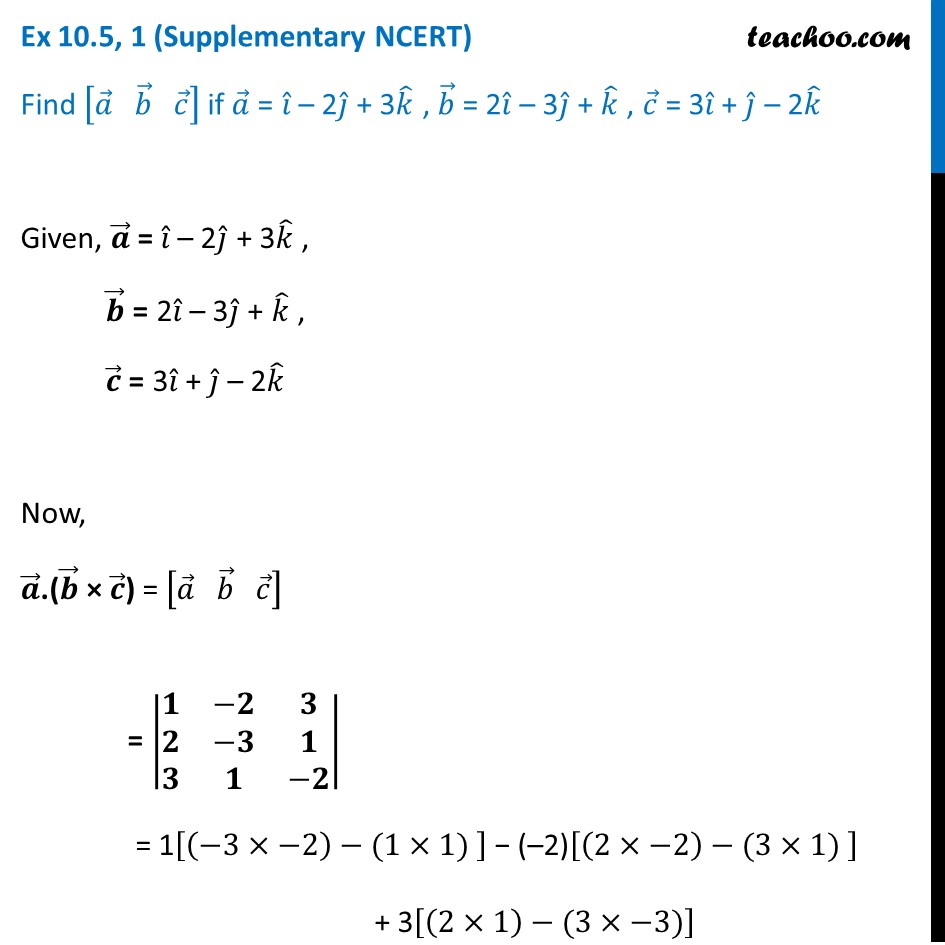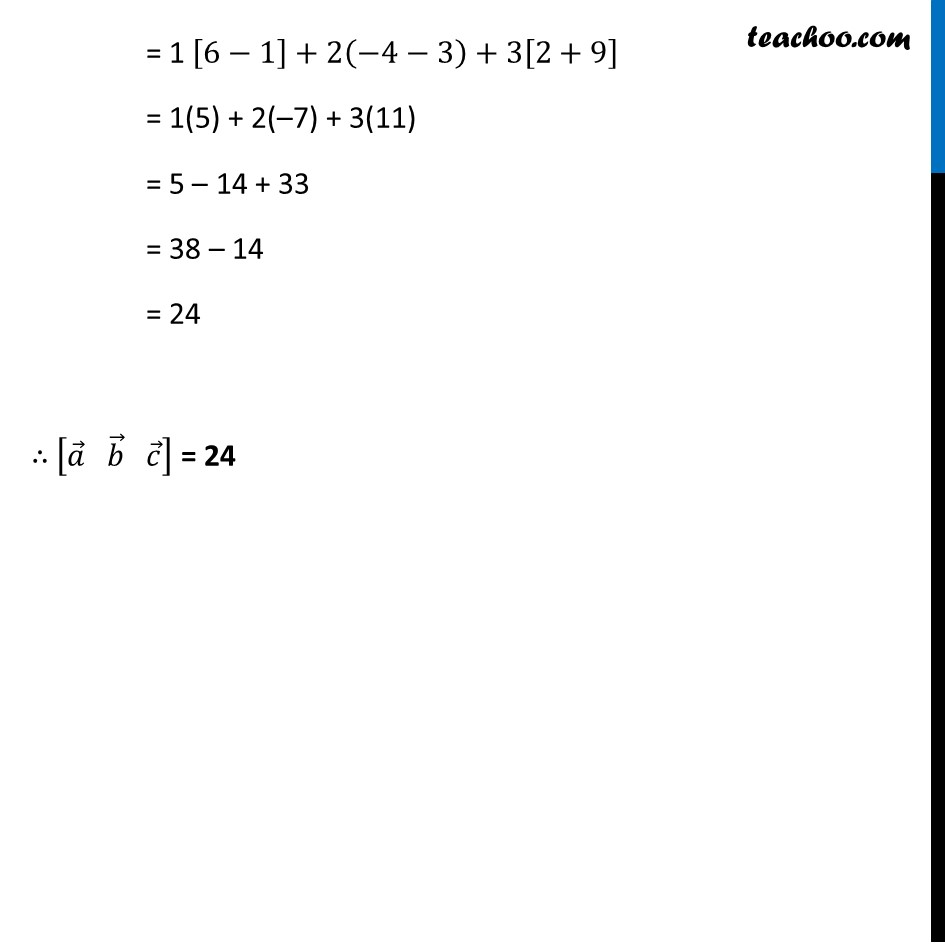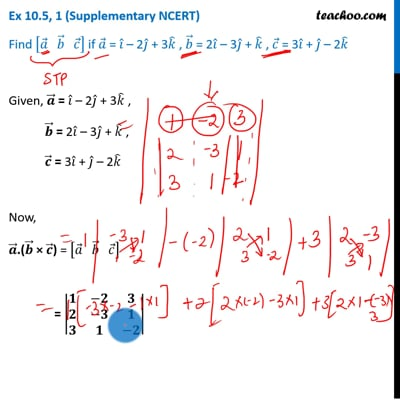Ex 10.5 (Supplementary NCERT)

Chapter 10 Class 12 Vector Algebra
Serial order wiseThis video is only available for Teachoo black users

Introducing your new favourite teacher - Teachoo Black, at only ₹83 per month

### Transcript

Ex 10.5, 1 (Supplementary NCERT) Find [𝑎 ⃗" " 𝑏 ⃗" " 𝑐 ⃗ ] if 𝑎 ⃗ = 𝑖 ̂ – 2𝑗 ̂ + 3𝑘 ̂ , 𝑏 ⃗ = 2𝑖 ̂ – 3𝑗 ̂ + 𝑘 ̂ , 𝑐 ⃗ = 3𝑖 ̂ + 𝑗 ̂ – 2𝑘 ̂Given, 𝒂 ⃗ = 𝑖 ̂ – 2𝑗 ̂ + 3𝑘 ̂ , 𝒃 ⃗ = 2𝑖 ̂ – 3𝑗 ̂ + 𝑘 ̂ , 𝒄 ⃗ = 3𝑖 ̂ + 𝑗 ̂ – 2𝑘 ̂ Now, 𝒂 ⃗.(𝒃 ⃗ × 𝒄 ⃗) = [𝑎 ⃗" " 𝑏 ⃗" " 𝑐 ⃗ ] = |■8(𝟏&−𝟐&𝟑@𝟐&−𝟑&𝟏@𝟑&𝟏&−𝟐)| = 1[(−3×−2)−(1×1) ] − (–2)[(2×−2)−(3×1) ] + 3[(2×1)−(3×−3)] = 1 [6−1]+2(−4−3)+3[2+9] = 1(5) + 2(–7) + 3(11) = 5 – 14 + 33 = 38 – 14 = 24 ∴ [𝑎 ⃗" " 𝑏 ⃗" " 𝑐 ⃗ ] = 24